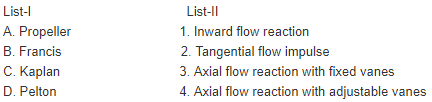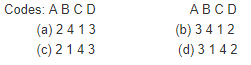Courses

# Hydraulic Turbine, Buoyancy And Flotation - MCQ Test 1

## 20 Questions MCQ Test Mock Test Series - Mechanical Engineering (ME) for GATE 2020 | Hydraulic Turbine, Buoyancy And Flotation - MCQ Test 1

Description
This mock test of Hydraulic Turbine, Buoyancy And Flotation - MCQ Test 1 for Mechanical Engineering helps you for every Mechanical Engineering entrance exam. This contains 20 Multiple Choice Questions for Mechanical Engineering Hydraulic Turbine, Buoyancy And Flotation - MCQ Test 1 (mcq) to study with solutions a complete question bank. The solved questions answers in this Hydraulic Turbine, Buoyancy And Flotation - MCQ Test 1 quiz give you a good mix of easy questions and tough questions. Mechanical Engineering students definitely take this Hydraulic Turbine, Buoyancy And Flotation - MCQ Test 1 exercise for a better result in the exam. You can find other Hydraulic Turbine, Buoyancy And Flotation - MCQ Test 1 extra questions, long questions & short questions for Mechanical Engineering on EduRev as well by searching above.
QUESTION: 1

### In a Pelton wheel, the bucket peripheral speed is 10 m/s, the water jetvelocity is 25 m/s and volumetric flow rate of the jet is 0.1m3/s. If the jetdeflection angle is120° and the flow is ideal, the power developed is:

Solution:

Ans. (c) From velocity triangle, Power developed = ∫ Q(Vw1+ Vw2) × u = 22.5 KW

QUESTION: 2

Solution:

Ans. (b)

QUESTION: 3

### Cavitation in a hydraulic turbine is most likely to occur at the turbine

Solution:

Ans. (d)

QUESTION: 4

Of all the power plants, hydel is more disadvantageous when one compares the

Solution:

Ans. (a) Of all the power plants, hydel is more disadvantageous when one compares the nearness to load centre because it is in hilly areas.

QUESTION: 5

If H is the head available for a hydraulic turbine, the power, speed and discharge, respectively are proportional to:

Solution:

Ans. (b)

QUESTION: 6

Consider the following statements:
A water turbine governor
1. Helps in starting and shutting down the turbo unit
2. Controls the speed of turbine set to match it with the hydroelectric system
3. Sets the amount of load which a turbine unit has to carry
Which of these statements are correct?

Solution:

Ans. (a)

QUESTION: 7

Match List-I with List-II and select the correct answer using the codes given below the lists:
List-I
A. Pelton turbine
B. Francis turbine
C. Propeller turbine
D. Kaplan turbine
List-II
1. Specific speed from 300 to 1000 axial flow with fixed runner vanes
2. Specific speed from 10 to 50 Tangential flow
3. Specific speed from 60 to 300 mixed flow
4. Specific speed from 300 to 1000 axial flow with adjustable runner vanes
Codes: A B C D                             A B C D
(a) 2 1 3 4                        (b) 4 1 3 2
(c) 2 3 1 4                        (d) 4 3 1 2

Solution:

Ans. (c)

QUESTION: 8

Match List-I (Types of turbines), List-II (Characteristics of turbines) and select the correct answer.Solution:

Ans. (d)

QUESTION: 9

Consider the following statements:
1. Pelton wheel is a tangential flow impulse turbine
2. Francis turbine is an axial flow reaction turbine
3. Kaplan turbine is a radial flow reaction turbine
Which of the above statements is/ are correct?

Solution:

Ans. (b)

QUESTION: 10

Which one of the following turbines is used in under water powerstations?

Solution:

Ans. (c)

QUESTION: 11

A Pelton wheel is ideally suited for

Solution:

Ans. (a)

QUESTION: 12

Which of the following types of turbine is/are suitable for tidal power plants?
1. Tubular turbine
2. Kaplan turbine
3. Bulb turbine
4. Francis turbine
Select the correct answer using the code given below:

Solution:

Ans. (b) See theory of bulb and tubular turbine in the theory section.

QUESTION: 13

The maximum number of jets generally employed in an impulse turbine without jet interference is:

Solution:

Ans. (b)

QUESTION: 14

Assertion (A): If a boat, built with sheet metal on wooden frame,has an average density which is greater than that of water, then the boat can float in water with its hollow face upward but will sink once it overturns
Reason (R): Buoyant force always acts in the upward direction.

Solution:

Ans. (b) Both A and R are true, but R does not give sufficient explanation for phenomenon at A. Location of Metacentre and centre of buoyancy decide about floating of a body.

QUESTION: 15

the Meta centre of a floating body, the body will be in stable equilibrium if

Solution:

Ans. (d)

QUESTION: 16

The metacentric height of a passenger ship is kept lower than that of a naval or a cargo ship because

Solution:

Ans. (c)

QUESTION: 17

A cylindrical vessel having its height equal to its diameter isfilled with liquid and moved horizontally at acceleration equal to acceleration due to gravity. The ratio of the liquid left in the vessel to the liquid at static equilibrium condition is:

Solution:

Ans. (c)

QUESTION: 18

Stability of a floating body can be improved by which of the following?
1. Making its width large
2. Making the draft small
3. Keeping the centre of mass low
4. Reducing its density
Select the correct answer using the code given below:

Solution:

Ans. (b) Stability of a floating body can be improved by making width large which will increase. I and will thus increase the metacentric height and keeping the centre of mass low and making the draft small.

QUESTION: 19

Consider the following statements:
Filling up a part of the empty hold of a ship with ballasts will
1. Reduce the metacentric height.
2. Lower the position of the centre of gravity.
3. Elevate the position of centre of gravity.
4. Elevate the position of centre of buoyancy.
Of these statements

Solution:

Ans. (d) Making bottom heavy lowers the c.g. of ship. It also increases displaced volume of water and thus the centre of displaced water, i.e. centre of buoyancy is elevated.

QUESTION: 20

Assertion (A): For a vertically immersed surface, the depth of the centre of pressure is independent of the density of the liquid.

Reason (R): Centre of pressure lies above the centre of area of the immersed surface.

Solution:

Ans. (c)